# Seaful Calendar 2024 now available!

Ocean Wildlife from around the world. Photos kindly donated by James Appleton, with all proceeds directly funding our work. Calendars are £12 each plus £3.50 postage, and will be posted out w/c 11th December, to arrive before Christmas.

` `

`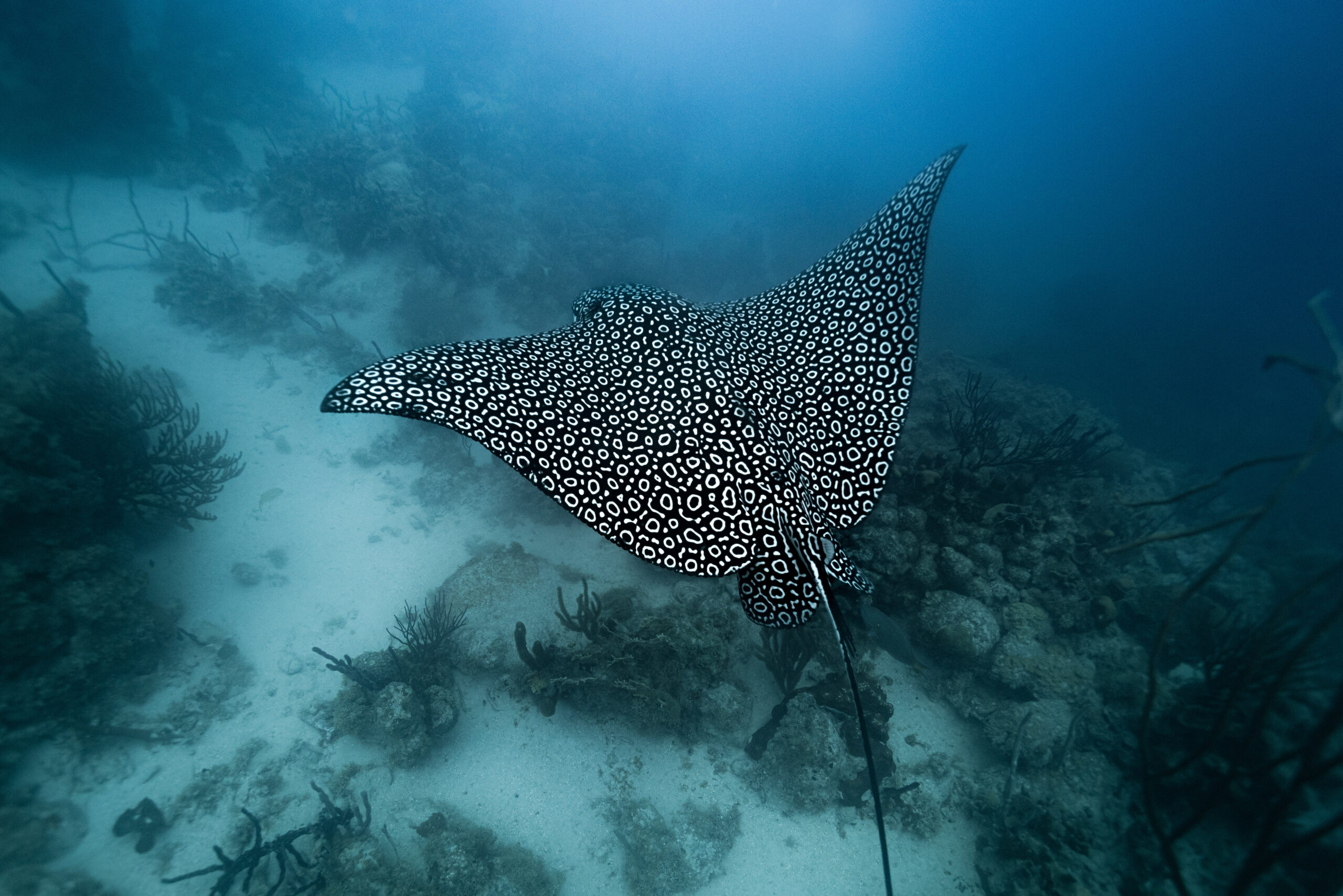`
`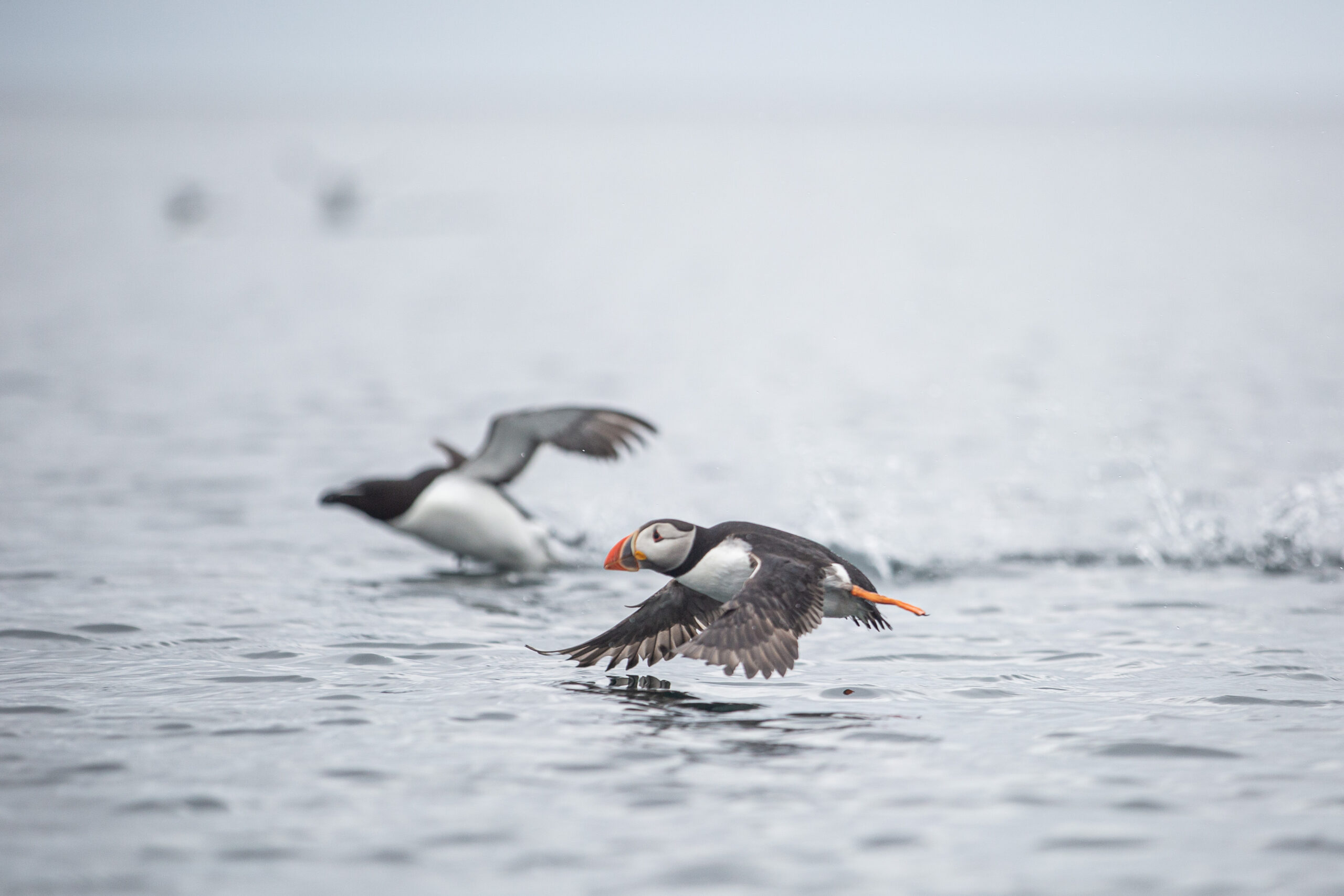`
`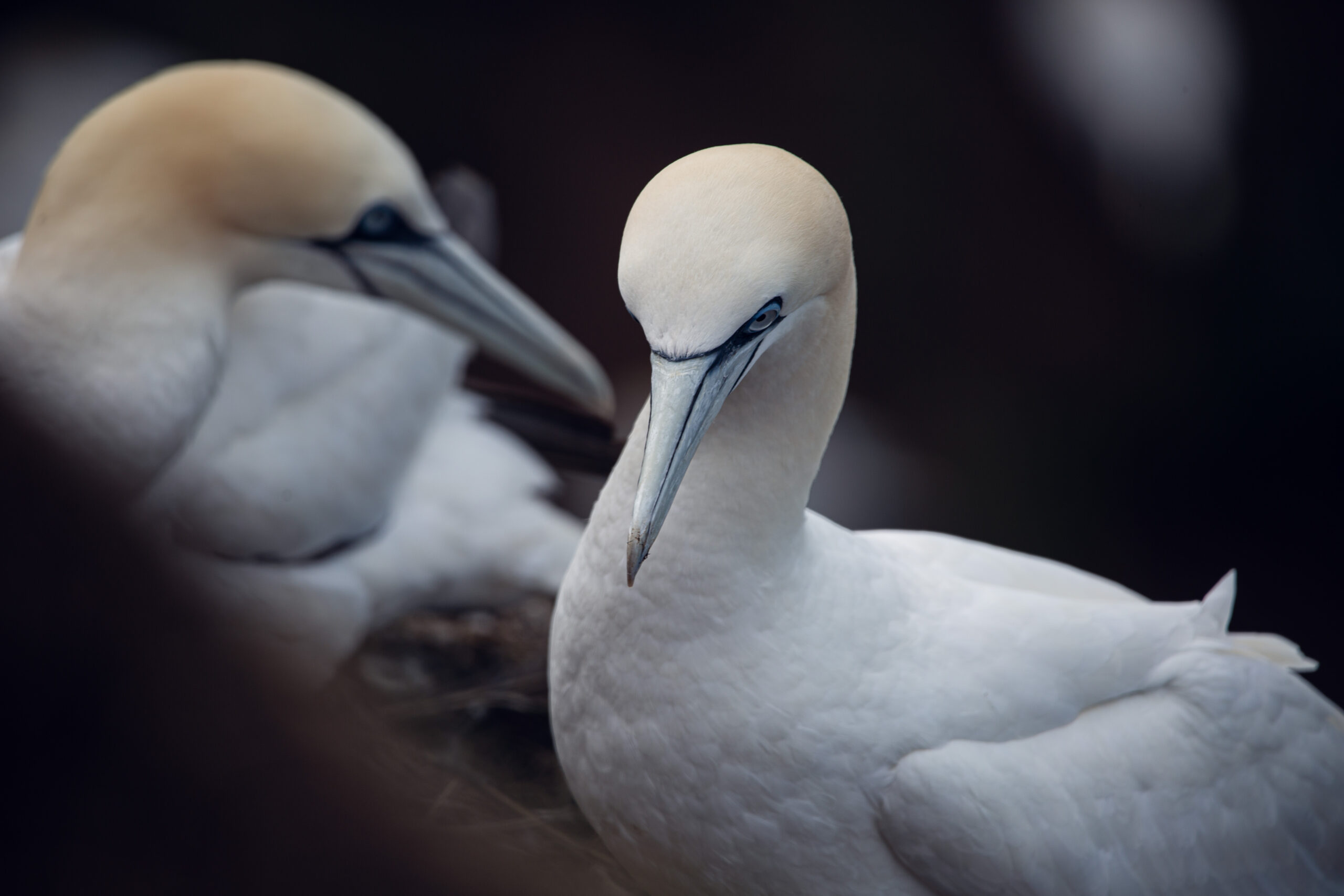`
`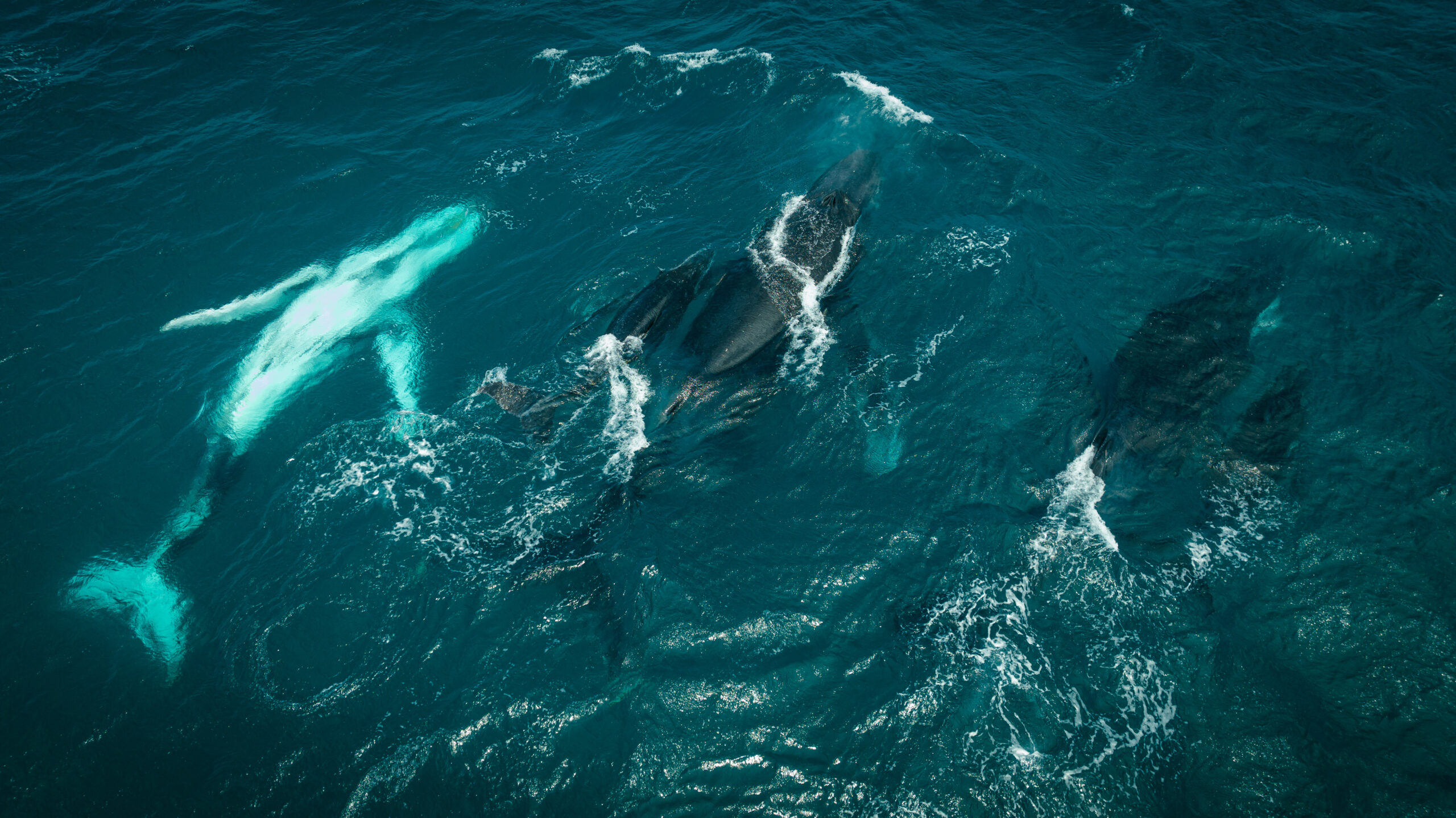`
`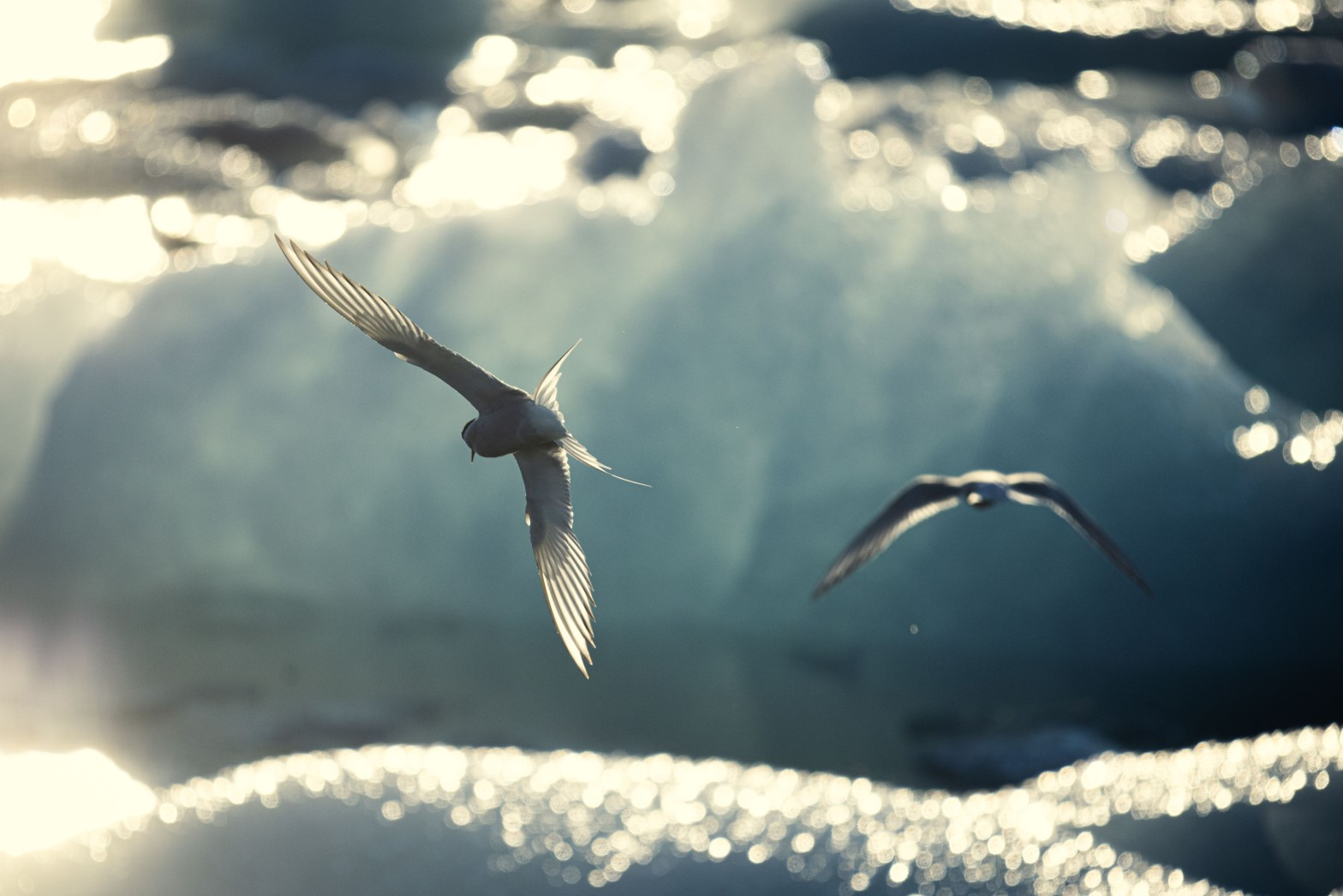`
``

## ` Ways to donate`

`Thank you so much for your kind donation. As a small charity, your donation will make a big difference.`

`If you would like to donate via a different method, please get in touch.`

``

``` (function(d, s, id) { var js, fjs = d.getElementsByTagName(s); if (d.getElementById(id)) return; js = d.createElement(s); js.id = id; js.src = "//connect.facebook.net/en_US/sdk.js#xfbml=1&version=v2.5"; fjs.parentNode.insertBefore(js, fjs); }(document, 'script', 'facebook-jssdk')); window.___gcfg = { lang: 'en-US' }; (function() { var po = document.createElement('script'); po.type = 'text/javascript'; po.async = true; po.src = 'https://apis.google.com/js/plusone.js'; var s = document.getElementsByTagName('script'); s.parentNode.insertBefore(po, s); })(); window.addEventListener('sfsi_functions_loaded', function() { if (typeof sfsi_responsive_toggle == 'function') { sfsi_responsive_toggle(0); // console.log('sfsi_responsive_toggle'); } }) window.addEventListener('sfsi_functions_loaded', function() { if (typeof sfsi_plugin_version == 'function') { sfsi_plugin_version(2.77); } }); function sfsi_processfurther(ref) { var feed_id = ''; var feedtype = 8; var email = jQuery(ref).find('input[name="email"]').val(); var filter = /^([a-zA-Z0-9_\.\-])+\@(([a-zA-Z0-9\-])+\.)+([a-zA-Z0-9]{2,4})+\$/; if ((email != "Enter your email") && (filter.test(email))) { if (feedtype == "8") { var url = "https://api.follow.it/subscription-form/" + feed_id + "/" + feedtype; window.open(url, "popupwindow", "scrollbars=yes,width=1080,height=760"); return true; } } else { alert("Please enter email address"); jQuery(ref).find('input[name="email"]').focus(); return false; } } var wpcf7 = {"api":{"root":"https:\/\/seaful.org.uk\/wp-json\/","namespace":"contact-form-7\/v1"},"cached":"1"}; var sfsi_icon_ajax_object = {"nonce":"448062cb67","ajax_url":"https:\/\/seaful.org.uk\/wp\/wp-admin\/admin-ajax.php","plugin_url":"https:\/\/seaful.org.uk\/app\/plugins\/ultimate-social-media-icons\/"}; !function(e){function r(r){for(var n,l,f=r,i=r,a=r,c=0,s=[];c<f.length;c++)l=f[c],Object.prototype.hasOwnProperty.call(o,l)&&o[l]&&s.push(o[l]),o[l]=0;for(n in i)Object.prototype.hasOwnProperty.call(i,n)&&(e[n]=i[n]);for(p&&p(r);s.length;)s.shift()();return u.push.apply(u,a||[]),t()}function t(){for(var e,r=0;r<u.length;r++){for(var t=u[r],n=!0,f=1;f<t.length;f++){var i=t[f];0!==o[i]&&(n=!1)}n&&(u.splice(r--,1),e=l(l.s=t))}return e}var n={},o={1:0},u=[];function l(r){if(n[r])return n[r].exports;var t=n[r]={i:r,l:!1,exports:{}};return e[r].call(t.exports,t,t.exports,l),t.l=!0,t.exports}l.m=e,l.c=n,l.d=function(e,r,t){l.o(e,r)||Object.defineProperty(e,r,{enumerable:!0,get:t})},l.r=function(e){"undefined"!=typeof Symbol&&Symbol.toStringTag&&Object.defineProperty(e,Symbol.toStringTag,{value:"Module"}),Object.defineProperty(e,"__esModule",{value:!0})},l.t=function(e,r){if(1&r&&(e=l(e)),8&r)return e;if(4&r&&"object"==typeof e&&e&&e.__esModule)return e;var t=Object.create(null);if(l.r(t),Object.defineProperty(t,"default",{enumerable:!0,value:e}),2&r&&"string"!=typeof e)for(var n in e)l.d(t,n,function(r){return e[r]}.bind(null,n));return t},l.n=function(e){var r=e&&e.__esModule?function(){return e.default}:function(){return e};return l.d(r,"a",r),r},l.o=function(e,r){return Object.prototype.hasOwnProperty.call(e,r)},l.p="/";var f=window.webpackJsonp=window.webpackJsonp||[],i=f.push.bind(f);f.push=r,f=f.slice();for(var a=0;a<f.length;a++)r(f[a]);var p=i;t()}([]); ```